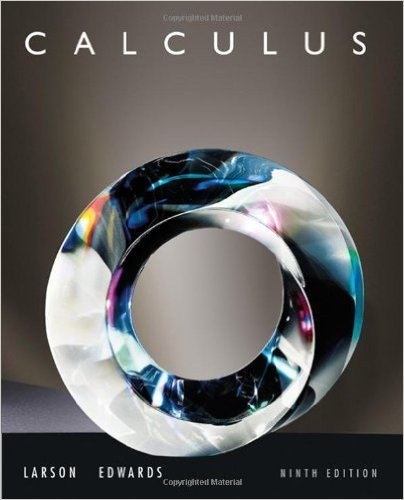×
×

# Solutions for Chapter 1.2: Finding Limits Graphically and Numerically## Full solutions for Calculus | 9th Edition

ISBN: 9780547167022Solutions for Chapter 1.2: Finding Limits Graphically and Numerically

Solutions for Chapter 1.2
4 5 0 285 Reviews
13
5
##### ISBN: 9780547167022

This textbook survival guide was created for the textbook: Calculus , edition: 9. This expansive textbook survival guide covers the following chapters and their solutions. Chapter 1.2: Finding Limits Graphically and Numerically includes 86 full step-by-step solutions. Calculus was written by and is associated to the ISBN: 9780547167022. Since 86 problems in chapter 1.2: Finding Limits Graphically and Numerically have been answered, more than 64108 students have viewed full step-by-step solutions from this chapter.

Key Calculus Terms and definitions covered in this textbook
• Absolute value of a real number

Denoted by |a|, represents the number a or the positive number -a if a < 0.

• Acute triangle

A triangle in which all angles measure less than 90°

• Base

See Exponential function, Logarithmic function, nth power of a.

• Cosecant

The function y = csc x

• Difference of complex numbers

(a + bi) - (c + di) = (a - c) + (b - d)i

• Direction angle of a vector

The angle that the vector makes with the positive x-axis

• Double-angle identity

An identity involving a trigonometric function of 2u

• Exponential decay function

Decay modeled by ƒ(x) = a ? bx, a > 0 with 0 < b < 1.

• Limit to growth

See Logistic growth function.

• Magnitude of an arrow

The magnitude of PQ is the distance between P and Q

• Piecewise-defined function

A function whose domain is divided into several parts with a different function rule applied to each part, p. 104.

• Placebo

In an experimental study, an inactive treatment that is equivalent to the active treatment in every respect except for the factor about which an inference is to be made. Subjects in a blind experiment do not know if they have been given the active treatment or the placebo.

• Right triangle

A triangle with a 90° angle.

• Row operations

See Elementary row operations.

• Solve algebraically

Use an algebraic method, including paper and pencil manipulation and obvious mental work, with no calculator or grapher use. When appropriate, the final exact solution may be approximated by a calculator

• Subtraction

a - b = a + (-b)

• Tangent line of ƒ at x = a

The line through (a, ƒ(a)) with slope ƒ'(a) provided ƒ'(a) exists.

• Terminal point

See Arrow.

• Trichotomy property

For real numbers a and b, exactly one of the following is true: a < b, a = b , or a > b.

• Xscl

The scale of the tick marks on the x-axis in a viewing window.

×